Latest Banking jobs   »   Quantitative Aptitude Quiz For IDBI AM/...

# Quantitative Aptitude Quiz For IDBI AM/ Bank of India PO 2023-26th March

Directions (1-5): What will come in place of the question mark (?) in each of the following series?

Q1. 64, ?, 48, 120, 420, 1890
(a) 27
(b) 32
(c) 30
(d) 24
(e) 36

Q2. 7, 5, ?, 17, 63, 309
(a) 10
(b) 3
(c) 8
(d) 7
(e) 9

Q3. 2, 7, 19, 38, 64, ?
(a) 128
(b) 112
(c) 97
(d) 88
(e) 92

Q4. 2, ?, 35, 98, 222, 437
(a) 9
(b) 17
(c) 15
(d) 8
(e) 7

Q5. 4, 8, 17, ?, 58, 94
(a) 38
(b) 27
(c) 29
(d) 37
(e) 33

Q6. A sum is lent at p% per annum simple interest for two years, Instead, had it been lent at 2p% per annum simple interest for q more years, than the interest would have been five times the earlier interest. Find the value of ‘q’?
(a) 5
(b) 2
(c) 3
(d) 1
(e) 4

Q7. 100 kgs of fruits are purchased for Rs. 1385. It is sold in such a way that after selling the whole quantity, the value of loss is equal to 37½% more than the amount obtained by selling 28 kgs of fruits. What is the selling price per kg.
(a) Rs. 10½
(b) Rs. 8½
(c) Rs. 8
(d) Rs. 12
(e) Rs. 10

Q8. Mixture of two containers A and B of equal capacity containing wine and water in the ratio 5 : 4 and 3 : 7 respectively are poured into another vessel C . If quantity of water in vessel C is 206 liter then find initial quantity of wine in vessel A.
(a) 110 L
(b) 95 L
(c) 90 L
(d) 100 L
(e) 80 L

Q9. A can do a work in x days and B can complete the same work in (x + 4) day. If A is 25% more efficient than B, then find the time in which A and B together can complete the whole work working alternatively on each day starting from A.
(a) 17¾ days
(b) 15⅔ days
(c) 18¾ days
(d) 20½ days
(e) 16¾ days

Q10. Average age of A, B and C is 40 years. Sum of present age of A and B is equal to present age of C. If ratio of age of A to C is 2 : 3 then find the difference in present age of B and C
(a) 25 year
(b) 30 year
(c) 20 year
(d) 40 year
(e) 50 year

Directions (11-15): Data given below shows number of girls and boys in two different classes in a school. Study the data carefully and answer the following question.
No. of boys in class ‘X’ is equal to number of girls in class ‘Y’. Number of boys in class ‘Y’ is 10 more than that of boys in class ‘X’. Ratio between number of girls in class ‘X’ to class ‘Y’ is 1 : 2. Total number of students in class ‘Y’ is 40% more than that in class ‘X’.
→ No. of mentors in class ‘X’ = 40% of boys in class ‘X’
→ No. of mentors in class ‘Y’ = 60% of girls in class ‘Y’

Q11. Total number of boys in class ‘Y’ is what percent more than total number of girls in class X ?
(a) 120%
(b) 220%
(c) 20%
(d) 60%
(e) 160%

Q12. Total number of mentors in class ‘Y’ is how much more than that in class ‘X’.
(a) 20
(b) 40
(c) 60
(d) 80
(e) 100

Q13. Boys in class ‘Z’ is 40% more than boys in class ‘Y’ while girls in class ‘Z’ is 20% more than girls in class ‘X’. Find total number of students in class ‘Z’.
(a) 284
(b) 194
(c) 224
(d) 204
(e) 214

Q14. Total number of boys in class X and Y together is how much more than total number of girls in class X and Y together.
(a) 180
(b) 120
(c) 90
(d) 30
(e) 60

Q15. Total girls passed in class ‘X’ and class ‘Y’ are 20% and 45% respectively. Find total number of girls who failed?
(a) 105
(b) 85
(c) 95
(d) 115
(e) 125

Solutions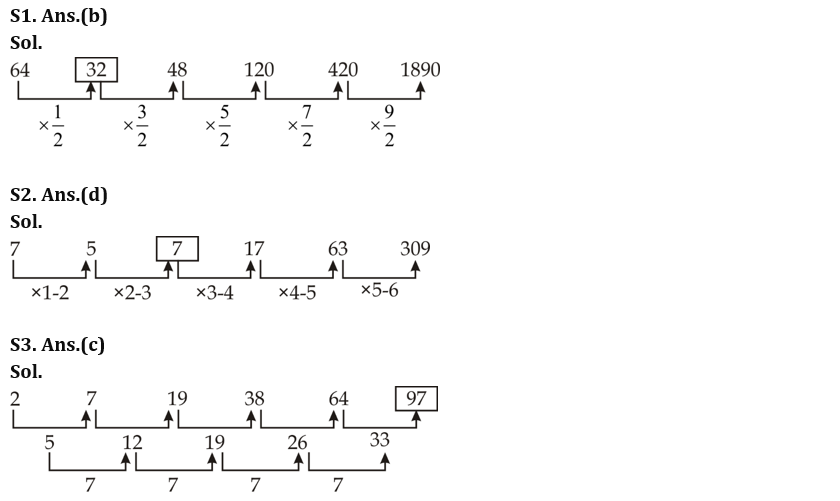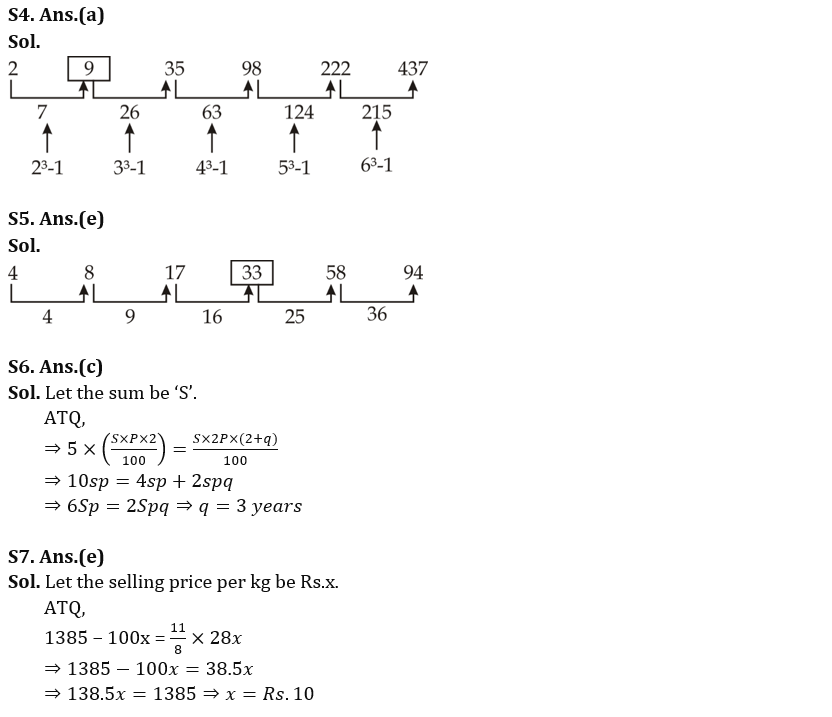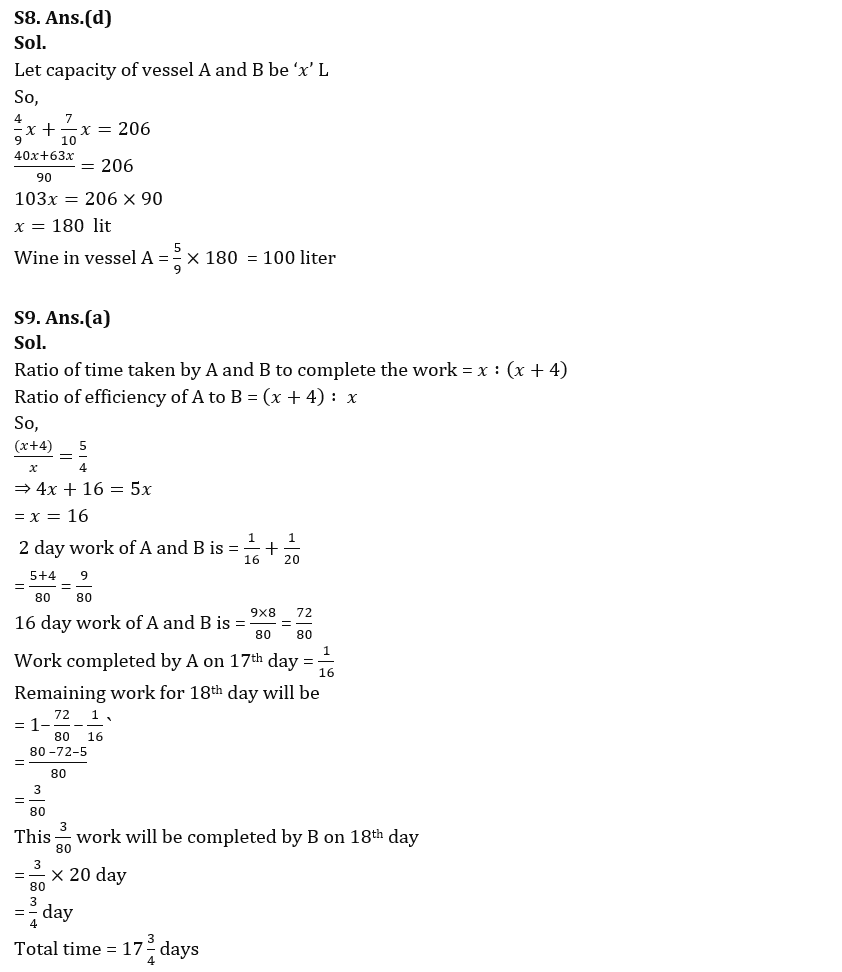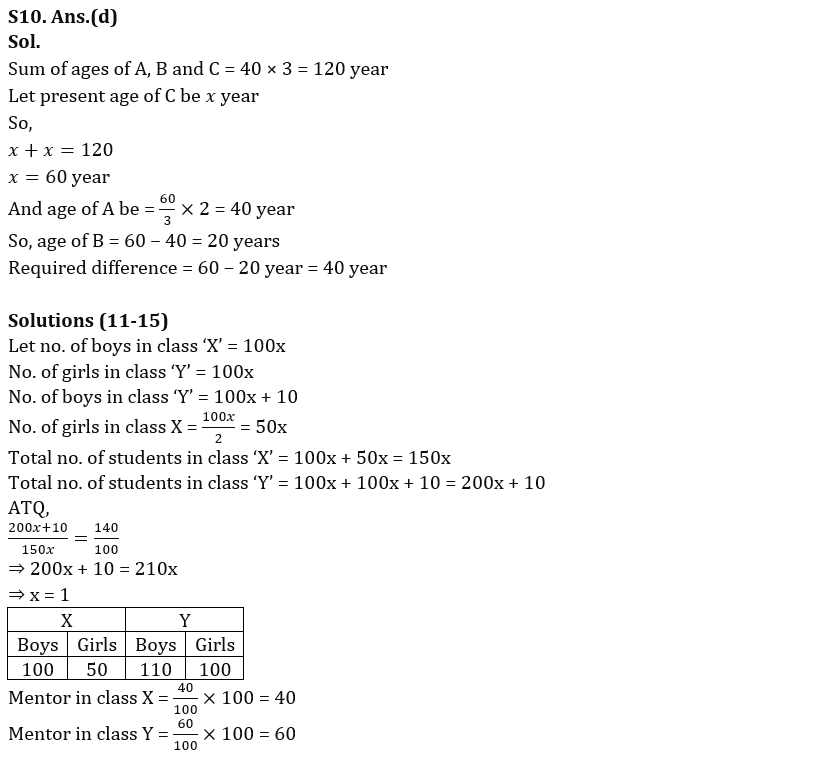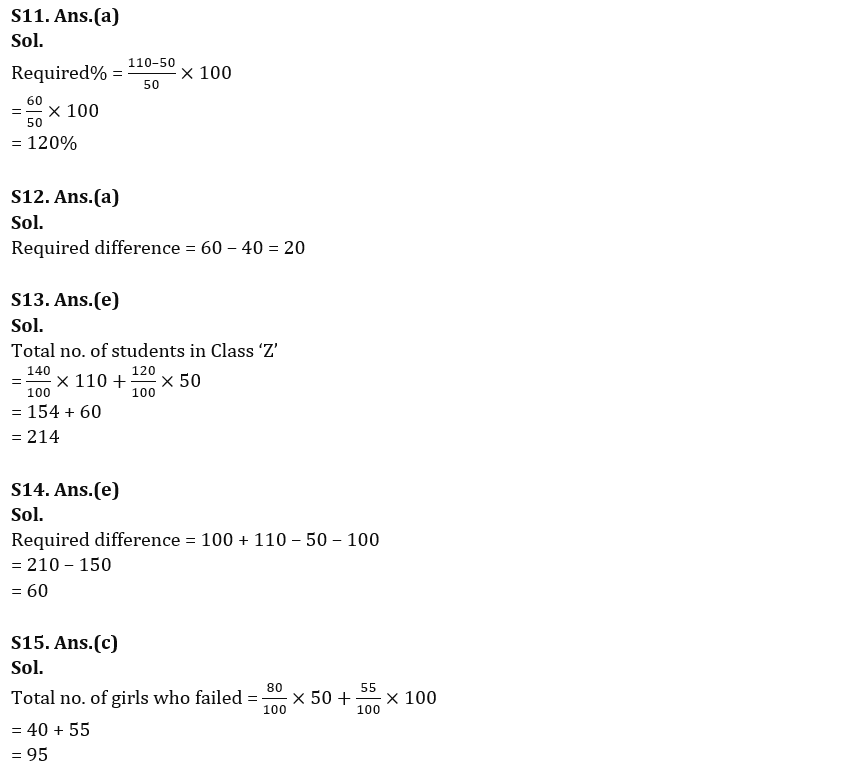## FAQs

### What is the selection process of the IDBI AM?

The selection process of IDBI AM is Online exam and Interview.

#### Congratulations!Union Budget 2023-24: Free PDF